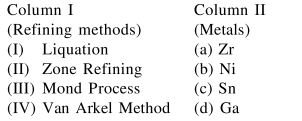Deepak Scored 45->99%ile with Bounce Back Crack Course. You can do it too!

# Match the refining methods (Column I) with metals (Column II).

Question:

Match the refining methods (Column I) with metals (Column II).1. (I) $-(\mathrm{b}) ;(\mathrm{II})-(\mathrm{c}) ;(\mathrm{III})-(\mathrm{d}) ;(\mathrm{IV})-(\mathrm{a})$

2. (I) $-(\mathrm{b}) ;(\mathrm{II})-(\mathrm{d}) ;(\mathrm{III})-(\mathrm{a}) ;(\mathrm{IV})-(\mathrm{c})$

3. $(\mathrm{I})-(\mathrm{c}) ;(\mathrm{II})-(\mathrm{a}) ;(\mathrm{III})-(\mathrm{b}) ;(\mathrm{IV})-(\mathrm{d})$

4. $(\mathrm{I})-(\mathrm{c}) ;(\mathrm{II})-(\mathrm{d}) ;(\mathrm{III})-(\mathrm{b}) ;(\mathrm{IV})-(\mathrm{a})$

Correct Option: , 4

Solution:

Liquation is used for Sn.

Zone refining is used for Ga.

Mond's process is used for $\mathrm{Ni}$.

Van arkel process is used for $\mathrm{Zr}$.